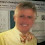## Saturday, May 28, 2011

### 75 Years of Computer Science

As Lipton and Felten note, today is the 75th anniversary of Turing's On Computable Numbers, With an Application to The Entscheidungsproblem, the seminal paper of seminal papers in computer science.

If you read any part of it, read Section 9, his justification of why the Turing Machine model captures computation, an argument that still resonates today.

No attempt has yet been made to show that the “computable” numbers include all numbers which would naturally be regarded as computable. All arguments which can be given are bound to be, fundamentally, appeals to intuition, and for this reason rather unsatisfactory mathematically. The real question at issue is “What are the possible processes which can be carried out in computing a number?”

The arguments which I shall use are of three kinds.

1. A direct appeal to intuition.
2. A proof of the equivalence of two definitions (in case the new definition has a greater intuitive appeal).
3. Giving examples of large classes of numbers which are computable.

Once it is granted that computable numbers are all “computable” several other propositions of the same character follow. In particular, it follows that, if there is a general process for determining whether a formula of the Hilbert function calculus is provable, then the determination can be carried out by a machine.

1.Does anyone know if Turing ever considered randomized computation? For example, arguing that randomized computation can be simulated by deterministic computation? Were there any randomized algorithms known 75 years ago? E.g. testing polynomial identity by evaluation in sufficiently many points?

2.I don't know about Turing, but randomized (numerical) algorithms date back at least to Metropolis and Ulam "The Monte Carlo Method", JASA 1949.

3.Rasmus: Yes. If you read Computing Machinery and Intelligence, Turing talks explicitly about randomized algorithms, and in a remarkably modern way.

4.I have often wished that Turing had soberly asked: “What are the verifiable processes which can be carried out in computing a number?” ...

... instead of Turing asking the fatefully harder question: “What are the possible processes which can be carried out in computing a number?”

The point being, that in our present-day world we understand very little about even so basic a class as P ... a class (AFAICT) whose membership and run-time ordering both are Turing-undecidable.

In the "sober-minded Turing" alternative universe, we might by now (with hard work and luck) have proved that verifiable-P (vP) and verifiable-NP (vNP) satisfy vP≠vNP ... whereas in our present universe we are not close at all (most folks think) to a proof that possible-P (pP) and possible-NP (pNP) satisfy pP≠pNP

Hmmm ... would a proof of vP≠vNP be satisfying to mathematicians who now have spent two generations attempting to prove pP≠pNP?

5.What are verifiable processes in this different question?

6.> What are verifiable processes in this different question?

I guess he means that the algorithm is not only correct but provably correct and not only polynomial-time but provably polynomial-time (conceivably, there could be polynomial-time algorithm for SAT that we cannot prove to be correct and we cannot prove to be polynomial-time). I am not sure if it makes any real difference.

7.Yes, by "verifiably correct" that is what I mean ... it was eye-opening to me to learn from TCS StackExchange .. via the question "Are runtime bounds in P decidable? (answer: no)" ... that verification procedures that we engineers take for granted—like "What is the runtime exponent for this code?"—are generically undecidable (these theorems go back to the work of Juris Hartmanis in the 1970s and 1980s).

This same topic arose on MathOverflow in association to Gil Kalai's topic "What notions are used but not clearly defined in modern mathematics?" specifically with regard to the question "What properties of P are not decidable in modern mathematics?"

The list of P's undecidable properties is surprisingly long, and contains some surprising members. For some purposes, would computer science be better off working with a restrictive subclass of P and a shorter list of undecidable properties?

8.To phrase the above question more formally (although I'm no expert), we know from the work of Juris Hartmanis (and from the explicit construction provided on TCS StackExchange by Emanuele Viola) that there exist Turing machines M whose runtime is in P, and yet the natural question "Is the runtime exponent of M less than a given value k?" is undecidable for these machines.

Then a similarly natural follow-on question, whose answer is (AFAICT) not known, is "Do there exist languages L that are recognized solely by those Turing machines in P whose runtime exponents are undecidable? And can examples of these machines and languages be finitely constructed?"

Needless to say, such Turing machines would be very interesting from a fundamental point-of-view, and concrete instantiations of them (or the provable infeasibility of constructing them) might be interesting too from a cryptographic point-of-view ... as examples of algorithms for which (by construction) natural questions like "How does this algorithm work?" or even verification questions like "Is this algorithm working correctly?" have no natural answers.

As for questions relating to the validation of sampling from distributions ... these questions seem to be even harder ... and yet they have considerable fundamental and practical importance.

Pointers to articles/monographs/textbooks discussing these issues would be very welcome ... Juris Hartmanis' monograph Feasible computations and provable complexity properties (1978) is the best I have found to date.

Very broadly speaking, these questions help us appreciate why so much of complexity theory is oracle-dependent. When Turing chose the fateful word "possible" instead of "verifable" in asking “What are the possible processes which can be carried out in computing a number?”, he implicitly embedded oracles into the foundations of computational complexity theory ... and thereby created great problems for mathematicians and engineers alike.